# Sum and Product of Graph

This is a continuation of the tutorial for the graph theory code which starts here. If you have not already installed and loaded the code from 'graph.spad' then you first need to follow the instructions on that page.

On this page we explore the methods for combining the graphs, these include:

• "+":(%,%) -> % Sum : disjoint union of nodes with arrows from appropriate input
• merge:(%,%) -> % Sum : union (not necessarily disjoint) of nodes with arrows merged in from appropriate input, if arrow exists from both inputs then it will be duplicated.
• "*":(%,%) -> GRPHPROD Tensor product : the tensor product G*H of graphs G and H is a graph such that the vertex set of G*H is the Cartesian product V(G) × V(H); and any two vertices (u,u') and (v,v') are adjacent in G × H if and only if u' is adjacent with v' and u is adjacent with v.
• cartesian:(%,%) -> GRPHPROD Cartesian product: the vertex set of G o H is the Cartesian product V(G) × V(H) and any two vertices (u,u') and (v,v') are adjacent in G o H if and only if either u = v and u' is adjacent with v' in H, or u' = v' and u is adjacent with v in G.
• ~ The complement or inverse of a graph.

### Simple Example

First we just construct a few types and macros to work with:

 ```(1) -> GS := DirectedGraph(String) (1) DirectedGraph(String) Type: Type (2) -> OBJT ==> Record(value:String,posX:NNI,posY:NNI) Type: Void (3) -> ARRW ==> Record(name:String,arrType:NNI,fromOb:NNI,_ toOb:NNI,xOffset:Integer,yOffset:Integer,map:List NNI) Type: Void```

First we will try out some sums and products of very simple graphs, just to get a feel for how things work.

Here we construct a very simple graph with two nodes "a" and "b" with an arrow between them:```(4) -> oba:OBJT := ["a",2,2] (4) [value= "a",posX= 2,posY= 2] Type: Record(value: String,posX: NonNegativeInteger,_ posY: NonNegativeInteger) (5) -> obb:OBJT := ["b",2,4] (5) [value= "b",posX= 2,posY= 4] Type: Record(value: String,posX: NonNegativeInteger,_ posY: NonNegativeInteger) (6) -> ara:ARRW := ["alpha",0,1,2,0,0,[]] (6) [name= "alpha",arrType= 0,fromOb= 1,_ toOb= 2,xOffset= 0,yOffset= 0,map= []] Type: Record(name: String,arrType: NonNegativeInteger,_ fromOb: NonNegativeInteger,toOb: NonNegativeInteger,_ xOffset: Integer,yOffset: Integer,map: List(NonNegativeInteger)) (7) -> hs1 := directedGraph([oba,obb],[ara])\$GS (7) "a","b"|"alpha":"a"->"b" Type: DirectedGraph(String) (8) -> diagramSvg("testGraph/testGraph4s1.svg",hs1,true) Type: Void```

Now we build the other operand for our various sums and products. This will also be a simple graph with two nodes and an arrow between them, but we will draw it differently and use different names to make the resulting diagrams more useful.```(9) -> ob1:OBJT := ["1",2,2] (9) [value= "1",posX= 2,posY= 2] Type: Record(value: String,posX: NonNegativeInteger,_ posY: NonNegativeInteger) (10) -> ob2:OBJT := ["2",4,2] (10) [value= "2",posX= 4,posY= 2] Type: Record(value: String,posX: NonNegativeInteger,_ posY: NonNegativeInteger) (11) -> ar1:ARRW := ["beta",0,1,2,0,0,[]] (11) [name= "beta",arrType= 0,fromOb= 1,toOb= 2,xOffset= 0,_ yOffset= 0,map= []] Type: Record(name: String,arrType: NonNegativeInteger,_ fromOb: NonNegativeInteger,toOb: NonNegativeInteger,_ xOffset: Integer,yOffset: Integer,map: List(NonNegativeInteger)) (12) -> hs2 := directedGraph([ob1,ob2],[ar1])\$GS (12) "1","2"|"beta":"1"->"2" Type: DirectedGraph(String) (13) -> diagramSvg("testGraph/testGraph4s2.svg",hs2,true) Type: Void```

Now we have two graphs to combine we can start testing out the sums and products. First a sum (which uses the "+" operator).

As you can see below this produces a graph with 4 vertices and 2 arrows. The arrows do not interact.```(14) -> hs3 := hs1+hs2 (14) "a","b","1","2"|"alpha":"a"->"b","beta":"1"->"2" Type: DirectedGraph(String) (15) -> diagramSvg("testGraph/testGraph4s3.svg",hs3,true) Type: Void```

We can now go on to to look at 'merge', this is similar to '+' above, in fact if we used the same operands as above it would have produced the same result. In order to illustrate the effect of 'merge' we will change the second operand slightly, it now has vertex 'a' and vertex '2' so it shares 'a' with the first operand. As we can see below, this common vertex is used by both arrows: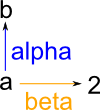```(16) -> hs4 := merge(hs1,directedGraph([oba,ob2],[ar1])\$GS) (16) "a","b","2"|"alpha":"a"->"b","beta":"a"->"2" Type: DirectedGraph(String) (17) -> diagramSvg("testGraph/testGraph4s4.svg",hs4,true) Type: Void```

We can now go on to look at the types of product supported. There are two types of product:

• Tensor Product
• Cartesian Product

First the tensor product, this generates 4 vertices (2*2) and one arrow which is a sort of composition of the two arrows from the two operands. The tensor product is represented by the '*' operation:```(18) -> hs5 := hs1*hs2 (18) ["a","1"],["a","2"],["b","1"],["b","2"]| "alpha*beta":["a","1"]->["b","2"] Type: DirectedGraph(Product(String,String)) (19) -> diagramSvg("testGraph/testGraph4s5.svg",hs5,true) Type: Void```

Now the Cartesian product, this always has the same vertices as the tensor product. However the arrows are different:```(20) -> hs6 := cartesian(hs1,hs2) (20) ["a","1"],["a","2"],["b","1"],["b","2"]|_ "beta#1":["a","1"]->["a","2"], "alpha#1":["a","1"]->["b","1"], "alpha#2":["a","2"]->["b","2"], "beta#2":["b","1"]->["b","2"] Type: DirectedGraph(Product(String,String)) (21) -> diagramSvg("testGraph/testGraph4s6.svg",hs6,true) Type: Void```

### Non-simple Example

Now that we have seen a simple case of these operations we will look at a more complicated case for the products. So that we can check our results we will use the examples on these wiki pages:

Here we construct the first operand from the wiki pages:```(22) -> obc:OBJT := ["a",3,2] (22) [value= "a",posX= 3,posY= 2] Type: Record(value: String,posX: NonNegativeInteger,_ posY: NonNegativeInteger) (23) -> obd:OBJT := ["b",2,4] (23) [value= "b",posX= 2,posY= 4] Type: Record(value: String,posX: NonNegativeInteger,_ posY: NonNegativeInteger) (24) -> obe:OBJT := ["c",4,6] (24) [value= "c",posX= 4,posY= 6] Type: Record(value: String,posX: NonNegativeInteger,_ posY: NonNegativeInteger) (25) -> obf:OBJT := ["d",3,8] (25) [value= "d",posX= 3,posY= 8] Type: Record(value: String,posX: NonNegativeInteger,_ posY: NonNegativeInteger) (26) -> obg:OBJT := ["e",3,10] (26) [value= "e",posX= 3,posY= 10] Type: Record(value: String,posX: NonNegativeInteger,_ posY: NonNegativeInteger) (27) -> arb:ARRW := ["alp1",0,1,2,0,0,[]] (27) [name= "alp1",arrType= 0,fromOb= 1,toOb= 2,_ xOffset= 0,yOffset= 0,map= []] Type: Record(name: String,arrType: NonNegativeInteger,_ fromOb: NonNegativeInteger,toOb: NonNegativeInteger,_ xOffset: Integer,yOffset: Integer,map: List(NonNegativeInteger)) (28) -> arc:ARRW := ["alp2",0,2,4,0,0,[]] (28) [name= "alp2",arrType= 0,fromOb= 2,toOb= 4,_ xOffset= 0,yOffset= 0,map= []] Type: Record(name: String,arrType: NonNegativeInteger,_ fromOb: NonNegativeInteger,toOb: NonNegativeInteger,_ xOffset: Integer,yOffset: Integer,map: List(NonNegativeInteger)) (29) -> ard:ARRW := ["alp3",0,1,3,0,0,[]] (29) [name= "alp3",arrType= 0,fromOb= 1,_ toOb= 3,xOffset= 0,yOffset= 0,map= []] Type: Record(name: String,arrType: NonNegativeInteger,_ fromOb: NonNegativeInteger,toOb: NonNegativeInteger,_ xOffset: Integer,yOffset: Integer,map: List(NonNegativeInteger)) (30) -> are:ARRW := ["alp4",0,3,4,0,0,[]] (30) [name= "alp4",arrType= 0,fromOb= 3,toOb= 4,xOffset= 0,_ yOffset= 0,map= []] Type: Record(name: String,arrType: NonNegativeInteger,_ fromOb: NonNegativeInteger,toOb: NonNegativeInteger,_ xOffset: Integer,yOffset: Integer,map: List(NonNegativeInteger)) (31) -> arf:ARRW := ["alp5",0,4,5,0,0,[]] (31) [name= "alp5",arrType= 0,fromOb= 4,toOb= 5,_ xOffset= 0,yOffset= 0,map= []] Type: Record(name: String,arrType: NonNegativeInteger,_ fromOb: NonNegativeInteger,toOb: NonNegativeInteger,_ xOffset: Integer,yOffset: Integer,map: List(NonNegativeInteger)) (32) -> hs11 := directedGraph([obc,obd,obe,obf,obg],_ [arb,arc,ard,are,arf])\$GS (32) "a","b","c","d","e"|"alp1":"a"->"b","alp2":"b"->"d", "alp3":"a"->"c","alp4":"c"->"d","alp5":"d"->"e" Type: DirectedGraph(String) (33) -> diagramSvg("testGraph/testGraph4s7.svg",hs11,true) Type: Void```

Now we build the other operand from the wiki pages.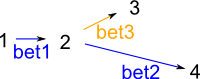```(34) -> ob3:OBJT := ["1",2,3] (34) [value= "1",posX= 2,posY= 3] Type: Record(value: String,posX: NonNegativeInteger,_ posY: NonNegativeInteger) (35) -> ob4:OBJT := ["2",4,3] (35) [value= "2",posX= 4,posY= 3] Type: Record(value: String,posX: NonNegativeInteger,_ posY: NonNegativeInteger) (36) -> ob5:OBJT := ["3",6,4] (36) [value= "3",posX= 6,posY= 4] Type: Record(value: String,posX: NonNegativeInteger,_ posY: NonNegativeInteger) (37) -> ob6:OBJT := ["4",8,2] (37) [value= "4",posX= 8,posY= 2] Type: Record(value: String,posX: NonNegativeInteger,_ posY: NonNegativeInteger) (38) -> ar2:ARRW := ["bet1",0,1,2,0,0,[]] (38) [name= "bet1",arrType= 0,fromOb= 1,toOb= 2,_ xOffset= 0,yOffset= 0,map= []] Type: Record(name: String,arrType: NonNegativeInteger,_ fromOb: NonNegativeInteger,toOb: NonNegativeInteger,_ xOffset: Integer,yOffset: Integer,map: List(NonNegativeInteger)) (39) -> ar3:ARRW := ["bet2",0,2,4,0,0,[]] (39) [name= "bet2",arrType= 0,fromOb= 2,toOb= 4,_ xOffset= 0,yOffset= 0,map= []] Type: Record(name: String,arrType: NonNegativeInteger,_ fromOb: NonNegativeInteger,toOb: NonNegativeInteger,_ xOffset: Integer,yOffset: Integer,map: List(NonNegativeInteger)) (40) -> ar4:ARRW := ["bet3",0,2,3,0,0,[]] (40) [name= "bet3",arrType= 0,fromOb= 2,_ toOb= 3,xOffset= 0,yOffset= 0,map= []] Type: Record(name: String,arrType: NonNegativeInteger,_ fromOb: NonNegativeInteger,toOb: NonNegativeInteger,_ xOffset: Integer,yOffset: Integer,map: List(NonNegativeInteger)) (41) -> hs12 := directedGraph([ob3,ob4,ob5,ob6],[ar2,ar3,ar4])\$GS (41) "1","2","3","4"|"bet1":"1"->"2","bet2":"2"->"4","bet3":"2"->"3" Type: DirectedGraph(String) (42) -> diagramSvg("testGraph/testGraph4s8.svg",hs12,true) Type: Void```

We can now go on to look at the types of product supported. There are two types of product:

• Tensor Product
• Cartesian Product

First the tensor product: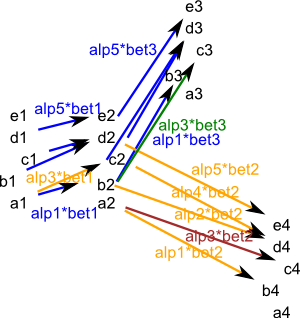```(43) -> hs13 := hs11*hs12 (43) ["a","1"],["a","2"],["a","3"],["a","4"],["b","1"],["b","2"],["b","3"], ["b","4"],["c","1"],["c","2"],["c","3"],["c","4"],["d","1"],["d","2"], ["d","3"],["d","4"],["e","1"],["e","2"],["e","3"],["e","4"] | "alp1*bet1":["a","1"]->["b","2"],"alp1*bet3":["a","2"]->["b","3"], "alp1*bet2":["a","2"]->["b","4"],"alp3*bet1":["a","1"]->["c","2"], "alp3*bet3":["a","2"]->["c","3"],"alp3*bet2":["a","2"]->["c","4"], "alp2*bet1":["b","1"]->["d","2"],"alp2*bet3":["b","2"]->["d","3"], "alp2*bet2":["b","2"]->["d","4"],"alp4*bet1":["c","1"]->["d","2"], "alp4*bet3":["c","2"]->["d","3"],"alp4*bet2":["c","2"]->["d","4"], "alp5*bet1":["d","1"]->["e","2"],"alp5*bet3":["d","2"]->["e","3"], "alp5*bet2":["d","2"]->["e","4"] Type: DirectedGraph(Product(String,String)) (44) -> diagramSvg("testGraph/testGraph4s9.svg",hs13,true) Type: Void```

Now the Cartesian product:```(45) -> hs14 := cartesian(hs11,hs12) (45) ["a","1"],["a","2"],["a","3"],["a","4"],["b","1"],["b","2"],["b","3"], ["b","4"],["c","1"],["c","2"],["c","3"],["c","4"],["d","1"],["d","2"], ["d","3"],["d","4"],["e","1"],["e","2"],["e","3"],["e","4"] | "bet1#1":["a","1"]->["a","2"],"bet3#1":["a","2"]->["a","3"], "bet2#1":["a","2"]->["a","4"],"alp1#1":["a","1"]->["b","1"], "alp1#2":["a","2"]->["b","2"],"alp1#3":["a","3"]->["b","3"], "alp1#4":["a","4"]->["b","4"],"alp3#1":["a","1"]->["c","1"], "alp3#2":["a","2"]->["c","2"],"alp3#3":["a","3"]->["c","3"], "alp3#4":["a","4"]->["c","4"],"bet1#2":["b","1"]->["b","2"], "bet3#2":["b","2"]->["b","3"],"bet2#2":["b","2"]->["b","4"], "alp2#1":["b","1"]->["d","1"],"alp2#2":["b","2"]->["d","2"], "alp2#3":["b","3"]->["d","3"],"alp2#4":["b","4"]->["d","4"], "bet1#3":["c","1"]->["c","2"],"bet3#3":["c","2"]->["c","3"], "bet2#3":["c","2"]->["c","4"],"alp4#1":["c","1"]->["d","1"], "alp4#2":["c","2"]->["d","2"],"alp4#3":["c","3"]->["d","3"], "alp4#4":["c","4"]->["d","4"],"bet1#4":["d","1"]->["d","2"], "bet3#4":["d","2"]->["d","3"],"bet2#4":["d","2"]->["d","4"], "alp5#1":["d","1"]->["e","1"],"alp5#2":["d","2"]->["e","2"], "alp5#3":["d","3"]->["e","3"],"alp5#4":["d","4"]->["e","4"], "bet1#5":["e","1"]->["e","2"],"bet3#5":["e","2"]->["e","3"], "bet2#5":["e","2"]->["e","4"] Type: DirectedGraph(Product(String,String)) (46) -> diagramSvg("testGraph/testGraph4s10.svg",hs14,true) Type: Void```

### Check Product Axioms

Here I would like to investigate the properties of the tensor and Cartesian products of graph. So is it associative? First we need to create 3 elements of graph which are not too big but just big enough to be interesting:

Here is the first graph:```(47) -> hs21 := ["a","b","c"]::GS (47) "a","b","c"|"a1":"a"->"b","a2":"b"->"c" Type: DirectedGraph(String) (48) -> diagramSvg("testGraph/testGraph4s12.svg",hs21,true) Type: Void```

Here is the second graph:```(49) -> hs22 := cycleClosed(["1","2","3"],"b")\$GS (49) "1","2","3"|"b1":"1"->"2","b2":"2"->"3","b3":"3"->"1" Type: DirectedGraph(String) (50) -> diagramSvg("testGraph/testGraph4s13.svg",hs22,true) Type: Void```

Here is the third graph:```(51) -> hs23 := ["x","y","z"]::GS (51) "x","y","z"|"a1":"x"->"y","a2":"y"->"z" Type: DirectedGraph(String) (52) -> diagramSvg("testGraph/testGraph4s14.svg",hs23,true) Type: Void```

First we will try tensor product.

Here is (hs21*hs22)*hs23:```(53) -> hs24 := closedTensor(closedTensor(hs21,hs22,concat)\$GS,hs23,concat)\$GS (53) "a1x","a1y","a1z","a2x","a2y","a2z","a3x","a3y","a3z","b1x","b1y", "b1z","b2x","b2y","b2z","b3x","b3y","b3z","c1x","c1y","c1z","c2x", "c2y","c2z","c3x","c3y","c3z" | "a1*b1*a1":"a1x"->"b2y","a1*b1*a2":"a1y"->"b2z", "a1*b2*a1":"a2x"->"b3y","a1*b2*a2":"a2y"->"b3z", "a1*b3*a1":"a3x"->"b1y","a1*b3*a2":"a3y"->"b1z", "a2*b1*a1":"b1x"->"c2y","a2*b1*a2":"b1y"->"c2z", "a2*b2*a1":"b2x"->"c3y","a2*b2*a2":"b2y"->"c3z", "a2*b3*a1":"b3x"->"c1y","a2*b3*a2":"b3y"->"c1z" Type: DirectedGraph(String) (54) -> diagramSvg("testGraph/testGraph4s15.svg",hs24,false) Type: Void```

and here is hs21*(hs22*hs23) :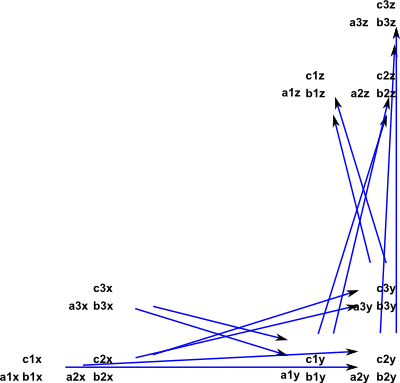```(55) -> hs25 := closedTensor(hs21,closedTensor(hs22,hs23,concat)\$GS,concat)\$GS (55) "a1x","a1y","a1z","a2x","a2y","a2z","a3x","a3y","a3z","b1x","b1y", "b1z","b2x","b2y","b2z","b3x","b3y","b3z","c1x","c1y","c1z","c2x", "c2y","c2z","c3x","c3y","c3z" | "a1*b1*a1":"a1x"->"b2y","a1*b1*a2":"a1y"->"b2z", "a1*b2*a1":"a2x"->"b3y","a1*b2*a2":"a2y"->"b3z", "a1*b3*a1":"a3x"->"b1y","a1*b3*a2":"a3y"->"b1z", "a2*b1*a1":"b1x"->"c2y","a2*b1*a2":"b1y"->"c2z", "a2*b2*a1":"b2x"->"c3y","a2*b2*a2":"b2y"->"c3z", "a2*b3*a1":"b3x"->"c1y","a2*b3*a2":"b3y"->"c1z" Type: DirectedGraph(String) (56) -> diagramSvg("testGraph/testGraph4s16.svg",hs25,false) Type: Void (57) -> looseEquals(hs24,hs25) (57) true Type: Boolean```

'looseEquals' returns true, so the two graphs are isomorphic, which means that (hs21*hs22)*hs23 is isomorphic to hs21*(hs22*hs23) where '*' is tensor product.

Now we can try Cartesian product.

Here is (hs21*hs22)*hs23: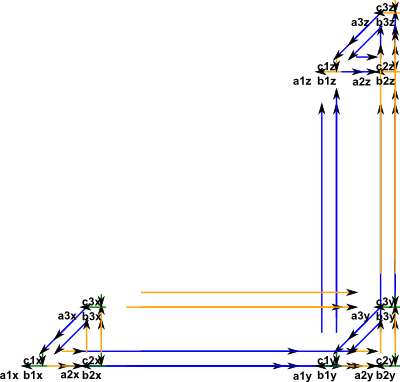```(58) -> hs26 := closedCartesian(closedCartesian(hs21,hs22,concat)\$GS,hs23,concat)\$GS (58) "a1x","a1y","a1z","a2x","a2y","a2z","a3x","a3y","a3z","b1x","b1y", "b1z","b2x","b2y","b2z","b3x","b3y","b3z","c1x","c1y","c1z","c2x", "c2y","c2z","c3x","c3y","c3z" | "a1#1":"a1x"->"a1y","a2#1":"a1y"->"a1z","b1#1#1":"a1x"->"a2x", "b1#1#2":"a1y"->"a2y","b1#1#3":"a1z"->"a2z","a1#1#1":"a1x"->"b1x", "a1#1#2":"a1y"->"b1y","a1#1#3":"a1z"->"b1z","a1#2":"a2x"->"a2y", "a2#2":"a2y"->"a2z","b2#1#1":"a2x"->"a3x","b2#1#2":"a2y"->"a3y", "b2#1#3":"a2z"->"a3z","a1#2#1":"a2x"->"b2x","a1#2#2":"a2y"->"b2y", "a1#2#3":"a2z"->"b2z","b3#1#1":"a3x"->"a1x","b3#1#2":"a3y"->"a1y", "b3#1#3":"a3z"->"a1z","a1#3":"a3x"->"a3y","a2#3":"a3y"->"a3z", "a1#3#1":"a3x"->"b3x","a1#3#2":"a3y"->"b3y","a1#3#3":"a3z"->"b3z", "a1#4":"b1x"->"b1y","a2#4":"b1y"->"b1z","b1#2#1":"b1x"->"b2x", "b1#2#2":"b1y"->"b2y","b1#2#3":"b1z"->"b2z","a2#1#1":"b1x"->"c1x", "a2#1#2":"b1y"->"c1y","a2#1#3":"b1z"->"c1z","a1#5":"b2x"->"b2y", "a2#5":"b2y"->"b2z","b2#2#1":"b2x"->"b3x","b2#2#2":"b2y"->"b3y", "b2#2#3":"b2z"->"b3z","a2#2#1":"b2x"->"c2x","a2#2#2":"b2y"->"c2y", "a2#2#3":"b2z"->"c2z","b3#2#1":"b3x"->"b1x","b3#2#2":"b3y"->"b1y", "b3#2#3":"b3z"->"b1z","a1#6":"b3x"->"b3y","a2#6":"b3y"->"b3z", "a2#3#1":"b3x"->"c3x","a2#3#2":"b3y"->"c3y","a2#3#3":"b3z"->"c3z", "a1#7":"c1x"->"c1y","a2#7":"c1y"->"c1z","b1#3#1":"c1x"->"c2x", "b1#3#2":"c1y"->"c2y","b1#3#3":"c1z"->"c2z","a1#8":"c2x"->"c2y", "a2#8":"c2y"->"c2z","b2#3#1":"c2x"->"c3x","b2#3#2":"c2y"->"c3y", "b2#3#3":"c2z"->"c3z","b3#3#1":"c3x"->"c1x","b3#3#2":"c3y"->"c1y", "b3#3#3":"c3z"->"c1z","a1#9":"c3x"->"c3y","a2#9":"c3y"->"c3z" Type: DirectedGraph(String) (59) -> diagramSvg("testGraph/testGraph4s17.svg",hs26,false) Type: Void```

and here is hs21*(hs22*hs23) :```(60) ->hs27 := closedCartesian(hs21,closedCartesian(hs22,hs23,concat)\$GS,concat)\$GS (60) "a1x","a1y","a1z","a2x","a2y","a2z","a3x","a3y","a3z","b1x","b1y", "b1z","b2x","b2y","b2z","b3x","b3y","b3z","c1x","c1y","c1z","c2x", "c2y","c2z","c3x","c3y","c3z" | "a1#1#1":"a1x"->"a1y","b1#1#1":"a1x"->"a2x","a2#1#1":"a1y"->"a1z", "b1#2#1":"a1y"->"a2y","b1#3#1":"a1z"->"a2z","a1#2#1":"a2x"->"a2y", "b2#1#1":"a2x"->"a3x","a2#2#1":"a2y"->"a2z","b2#2#1":"a2y"->"a3y", "b2#3#1":"a2z"->"a3z","b3#1#1":"a3x"->"a1x","a1#3#1":"a3x"->"a3y", "b3#2#1":"a3y"->"a1y","a2#3#1":"a3y"->"a3z","b3#3#1":"a3z"->"a1z", "a1#1":"a1x"->"b1x","a1#2":"a1y"->"b1y","a1#3":"a1z"->"b1z", "a1#4":"a2x"->"b2x","a1#5":"a2y"->"b2y","a1#6":"a2z"->"b2z", "a1#7":"a3x"->"b3x","a1#8":"a3y"->"b3y","a1#9":"a3z"->"b3z", "a1#1#2":"b1x"->"b1y","b1#1#2":"b1x"->"b2x","a2#1#2":"b1y"->"b1z", "b1#2#2":"b1y"->"b2y","b1#3#2":"b1z"->"b2z","a1#2#2":"b2x"->"b2y", "b2#1#2":"b2x"->"b3x","a2#2#2":"b2y"->"b2z","b2#2#2":"b2y"->"b3y", "b2#3#2":"b2z"->"b3z","b3#1#2":"b3x"->"b1x","a1#3#2":"b3x"->"b3y", "b3#2#2":"b3y"->"b1y","a2#3#2":"b3y"->"b3z","b3#3#2":"b3z"->"b1z", "a2#1":"b1x"->"c1x","a2#2":"b1y"->"c1y","a2#3":"b1z"->"c1z", "a2#4":"b2x"->"c2x","a2#5":"b2y"->"c2y","a2#6":"b2z"->"c2z", "a2#7":"b3x"->"c3x","a2#8":"b3y"->"c3y","a2#9":"b3z"->"c3z", "a1#1#3":"c1x"->"c1y" ,"b1#1#3":"c1x"->"c2x","a2#1#3":"c1y"->"c1z", "b1#2#3":"c1y"->"c2y","b1#3#3":"c1z"->"c2z","a1#2#3":"c2x"->"c2y", "b2#1#3":"c2x"->"c3x","a2#2#3":"c2y"->"c2z","b2#2#3":"c2y"->"c3y", "b2#3#3":"c2z"->"c3z","b3#1#3":"c3x"->"c1x","a1#3#3":"c3x"->"c3y", "b3#2#3":"c3y"->"c1y","a2#3#3":"c3y"->"c3z","b3#3#3":"c3z"->"c1z" Type: DirectedGraph(String) (61) -> diagramSvg("testGraph/testGraph4s18.svg",hs27,false) Type: Void (62) -> looseEquals(hs26,hs27) (62) false Type: Boolean ```

'looseEquals' returns false, however the diagrams look the same except some of the arrow names are different. So the graphs may well be isomorphic? We really need a proper test for isomorphism between graphs.

We can calculate the adjacency matrix of our required product by taking:

• Cartesian product: by taking Kronecker sum of adjacency matrix.
• Tensor product: by taking Kronecker product of adjacency matrix.
 ```(63) -> am21 := adjacencyMatrix(hs21) +0 0 0+ (63) |1 0 0| +0 1 0+ Type: Matrix(NonNegativeInteger) (64) -> am22 := adjacencyMatrix(hs22) +0 0 1+ (64) |1 0 0| +0 1 0+ Type: Matrix(NonNegativeInteger) (65) -> hs28 := closedTensor(hs21,hs22,concat)\$GS (65) "a1","a2","a3","b1","b2","b3","c1","c2","c3"|"a1*b1":"a1"->"b2", "a1*b2":"a2"->"b3", "a1*b3":"a3"->"b1", "a2*b1":"b1"->"c2", "a2*b2":"b2"->"c3", "a2*b3":"b3"->"c1" Type: DirectedGraph(String) (66) -> am28 := adjacencyMatrix(hs28) +0 0 0 0 0 0 0 0 0+ |0 0 0 0 0 0 0 0 0| |0 0 0 0 0 0 0 0 0| |0 0 1 0 0 0 0 0 0| (66) |1 0 0 0 0 0 0 0 0| |0 1 0 0 0 0 0 0 0| |0 0 0 0 0 1 0 0 0| |0 0 0 1 0 0 0 0 0| +0 0 0 0 1 0 0 0 0+ Type: Matrix(NonNegativeInteger) (67) -> kroneckerProduct(am21,am22) +0 0 0 0 0 0 0 0 0+ |0 0 0 0 0 0 0 0 0| |0 0 0 0 0 0 0 0 0| |0 0 1 0 0 0 0 0 0| (67) |1 0 0 0 0 0 0 0 0| |0 1 0 0 0 0 0 0 0| |0 0 0 0 0 1 0 0 0| |0 0 0 1 0 0 0 0 0| +0 0 0 0 1 0 0 0 0+ Type: Matrix(NonNegativeInteger) (68) -> hs29 := closedCartesian(hs21,hs22,concat)\$GS (68) "a1","a2","a3","b1","b2","b3","c1","c2","c3"|"b1#1":"a1"->"a2", "b2#1":"a2"->"a3","b3#1":"a3"->"a1","a1#1":"a1"->"b1", "a1#2":"a2"->"b2","a1#3":"a3"->"b3","b1#2":"b1"->"b2", "b2#2":"b2"->"b3","b3#2":"b3"->"b1","a2#1":"b1"->"c1", "a2#2":"b2"->"c2","a2#3":"b3"->"c3","b1#3":"c1"->"c2", "b2#3":"c2"->"c3","b3#3":"c3"->"c1" Type: DirectedGraph(String) (69) -> am29 := adjacencyMatrix(hs29) +0 0 1 0 0 0 0 0 0+ |1 0 0 0 0 0 0 0 0| |0 1 0 0 0 0 0 0 0| |1 0 0 0 0 1 0 0 0| (69) |0 1 0 1 0 0 0 0 0| |0 0 1 0 1 0 0 0 0| |0 0 0 1 0 0 0 0 1| |0 0 0 0 1 0 1 0 0| +0 0 0 0 0 1 0 1 0+ Type: Matrix(NonNegativeInteger) (70) -> kroneckerSum(am21,am22) +0 0 1 0 0 0 0 0 0+ |1 0 0 0 0 0 0 0 0| |0 1 0 0 0 0 0 0 0| |1 0 0 0 0 1 0 0 0| (70) |0 1 0 1 0 0 0 0 0| |0 0 1 0 1 0 0 0 0| |0 0 0 1 0 0 0 0 1| |0 0 0 0 1 0 1 0 0| +0 0 0 0 0 1 0 1 0+ Type: Matrix(NonNegativeInteger)```

That concludes our overview of ways to combine graphs such as sums and products graphs, click here to go to the next stage of the tutorial which is about ways to create new graphs by mapping from existing graphs.